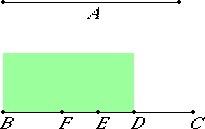# Proposition 18

If there are two unequal straight lines, and to the greater there is applied a parallelogram equal to the fourth part of the square on the less but falling short by a square, and if it divides it into incommensurable parts, then the square on the greater is greater than the square on the less by the square on a straight line incommensurable with the greater. And if the square on the greater is greater than the square on the less by the square on a straight line incommensurable with the greater, and if there is applied to the greater a parallelogram equal to the fourth part of the square on the less but falling short by a square, then it divides it into incommensurable parts.
X.17,Lemma

Let A and BC be two unequal straight lines, of which BC is the greater, and to BC let there be applied a parallelogram equal to the fourth part of the square on the less, A, but falling short by a square. Let this be the rectangle BD by DC, and let BD be incommensurable in length with DC.

I say that the square on BC is greater than the square on A by the square on a straight line incommensurable with BC.

With the same construction as before, we can prove similarly that the square on BC is greater than the square on A by the square on FD.

It is to be proved that BC is incommensurable in length with DF.X.16

Since BD is incommensurable in length with DC, therefore BC is also incommensurable in length with CD.

But DC is commensurable with the sum of BF and DC, therefore BC is incommensurable with the sum of BF and DC, so that BC is also incommensurable in length with the remainder FD.

And the square on BC is greater than the square on A by the square on FD, therefore the square on BC is greater than the square on A by the square on a straight line incommensurable with BC.

Next, let the square on BC be greater than the square on A by the square on a straight line incommensurable with BC. Apply to BC a parallelogram equal to the fourth part of the square on A but falling short by a square. Let this be the rectangle BD by DC.

It is to be proved that BD is incommensurable in length with DC.

With the same construction, we can prove similarly that the square on BC is greater than the square on A by the square on FD.

X.16

But the square on BC is greater than the square on A by the square on a straight line incommensurable with BC, therefore BC is incommensurable in length with FD, so that BC is also incommensurable with the remainder, the sum of BF and DC.

But the sum of BF and DC is commensurable in length with DC, therefore BC is also incommensurable in length with DC, so that, taken separately, BD is also incommensurable in length with DC.

Therefore, if there are two unequal straight lines, and to the greater there is applied a parallelogram equal to the fourth part of the square on the less but falling short by a square, and if it divides it into incommensurable parts, then the square on the greater is greater than the square on the less by the square on a straight line incommensurable with the greater. And if the square on the greater is greater than the square on the less by the square on a straight line incommensurable with the greater, and if there is applied to the greater a parallelogram equal to the fourth part of the square on the less but falling short by a square, then it divides it into incommensurable parts.

Q.E.D.

## Guide

This proposition is a logical variant of the last. It is used in frequently in Book X starting with X.33.